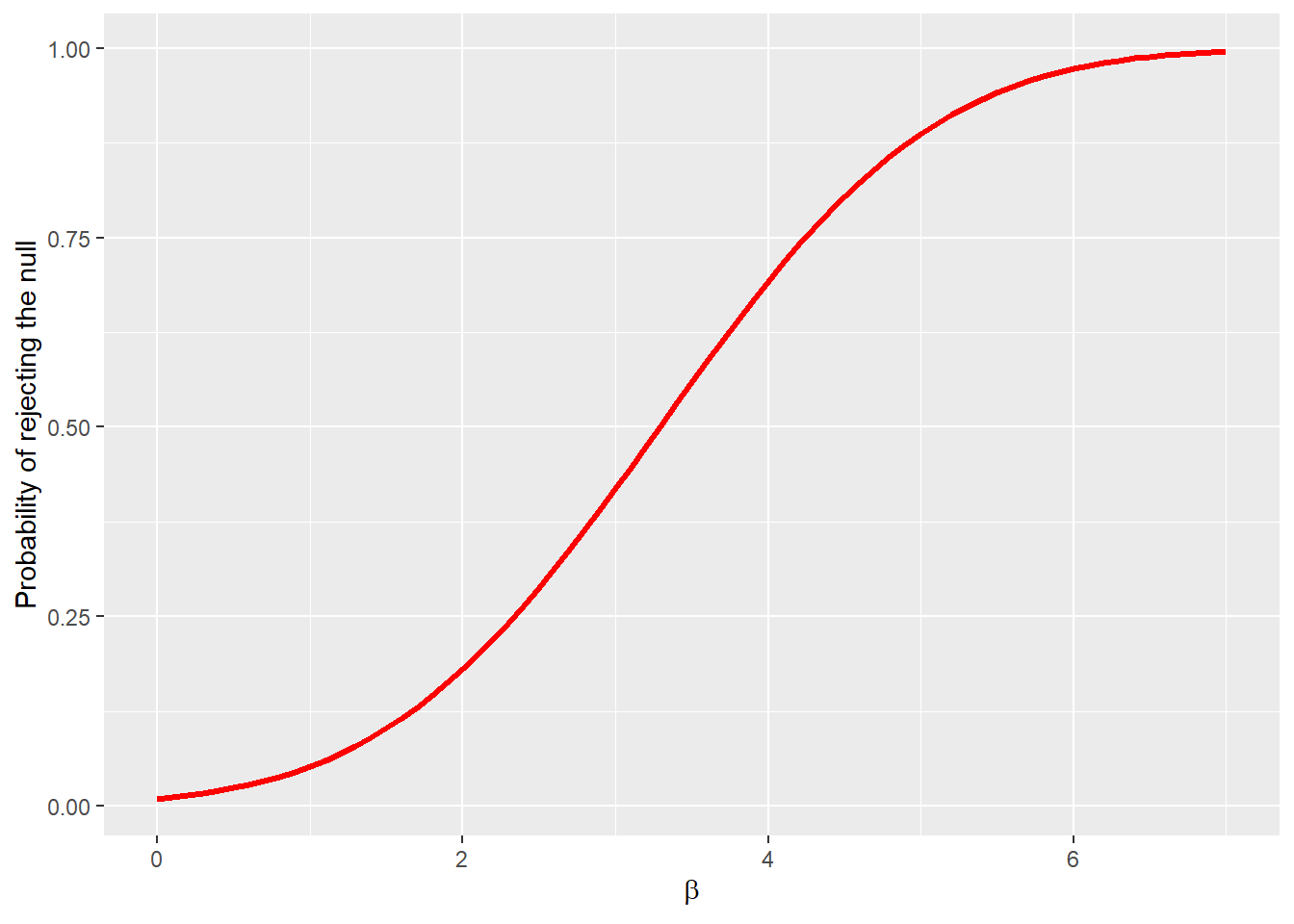# Chapter 6 Hypothesis Testing and Interval Estimation; Answering Research Questions

## 6.1 Computing Corner

We will learn the basisc for hypothesis testing in R.

### 6.1.1 Probability Distributions in R

For every probability distribution there are four commands. These command for each distribution are prepended by a letter to indicate the functionality.

• “d” returns the height of the probability “d”ensity function
• “p” returns the cummulative density function or the “p”robability of being being between two values of the random variable.
• “q” returns the inverse density function or the value of the random variable (“q”uantile) given a probability.
• “r” returns a “r”andomly generated number from the probability distribution

The distributions you are most likely to encounter in econometrics are the normal (norm), the F distribution (f), the chi-square distribution (chisq), and Student’s t-distribution (t). Others include the uniform (unif), binomial (binom), Poisson (pois), etc. Use of the help tab in the Files/Plots/Packages/Help pane or use of args will list the arguments necessary to extract value for each distribution.

### 6.1.2 Critical Values in R

To calculate critical values to perform a hypothesis test use the “q” version of the probability distribution. This will return the quantile for the given probability. The probability under the curve will be cummulative from $$-\infty$$ to the quantile returned. The “q” version will return the critical value for a one-tail test. Suppose you’d like to test the following hypothesis about $$\mu$$:

$H_0:\mu=0$ $H_1:\mu<0$ at the $$\alpha=.05$$ level of significance. To calculate the critical t-stastic call qt(p = .05, df = n-1). You know from args(qt) the default value of the argument lower.tail is TRUE. Suppose, instead, you’d like to test the following hypothesis about $$\mu$$

$H_0:\mu=0$ $H_1:\mu>0$ at the $$\alpha = .10$$ level of significance. You can call qt in two ways:

1. qt(p = .10, df = n-1, lower.tail = FALSE) or
2. qt(p = .90, df = n-1)

Finally, suppose you’d like to test the following hypothesis about $$\mu$$

$H_0:\mu=0$ $H_1:\mu\ne0$ at the $$\alpha=.01$$ level of significance. Since the t-distribution is symmetric you can use the lower tail or upper tail value and -1 times it. You can call qt in three ways:

1. qt(p = .005, df = n-1) or
2. qt(p = .005, df = n-1, lower.tail = FALSE) or
3. qt(p = .995, df = n-1)

You can find crtical values for the normal, F, and $$\chi^2$$ distributions with similar function calls.

#### 6.1.2.1p values in R

To calculate p values in R, use the “p” version of the distribution call. So suppose we test the following hypothesis:

$H_0:\sigma_1^2=\sigma_2^2$ $H_0:\sigma_1^2\ne\sigma_2^2$

at the $$\alpha=.05$$ level of significance. We could use an F test of the form

$F=\frac{s_x^2}{s_y^2}$

where $$s_x^2$$ and $$s_y^2$$ are the sample variances with n-1 and m-1 degrees of freedom. To calculate the p value, call pf(F, n-1, m-1) where F is the value calculated above.

### 6.1.3 Confidence Intervals for OLS estimates

In addition to confint(), confint_tidy() from the broom package will create a tibble of the low and high values for each estimate. The default level of confidence is 95%.

### 6.1.4 Power Curves

The power curve represents the probability of making Type II error under alternative null hypotheses. We can generate the power of the test with the pwr.norm.test(d = NULL, n = NULL, sig.level =.05, power = NULL, alternative = c("two-sided", "less", "greater")) call from the pwr package and plot the power with ggplot. To estimate the power we need the effect size $$d = \beta_i - \beta$$ where $$\beta$$ is the hypothesised paramater. We will use $H_0: \beta = 0$ $H_1: \beta > 0$

The $$\beta_i$$ represent alternative null hypothseses for $$\beta$$. Let’s let $$0 < beta < 7$$. Let the significance level be $$\alpha=.01$$ and $$se_{\beta} = 1$$.

library(tidyverse)
library(pwr)

beta_i <- seq(0, 7, .1)
se_beta <- 1 # to keep se_beta = 1 we will set n = 1 below.

pwr <- pwr.2p.test(beta_i, n = 1, sig.level = .01, alternative = "greater")

#the output is a list we need to extract, h and power from pwr

data <- tibble(beta = pwr$h, power = pwr$power)

data %>%
ggplot(aes(x = beta, y = power)) +
geom_line() +
ylab("Probability of rejecting the null") +
xlab(expression(beta))